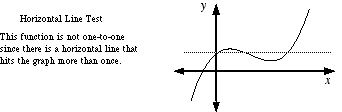# horizontal line test

Horizontal Line Test

A test use to determine if a function is one-to-one. If a horizontalline intersects a function's graph more than once, then the function is not one-to-one.

Note: The function y = f(x) is a function if it passes the vertical line test. It is a one-to-one function if it passes both the vertical line test and the horizontal line test.